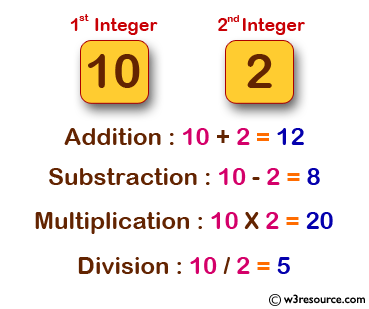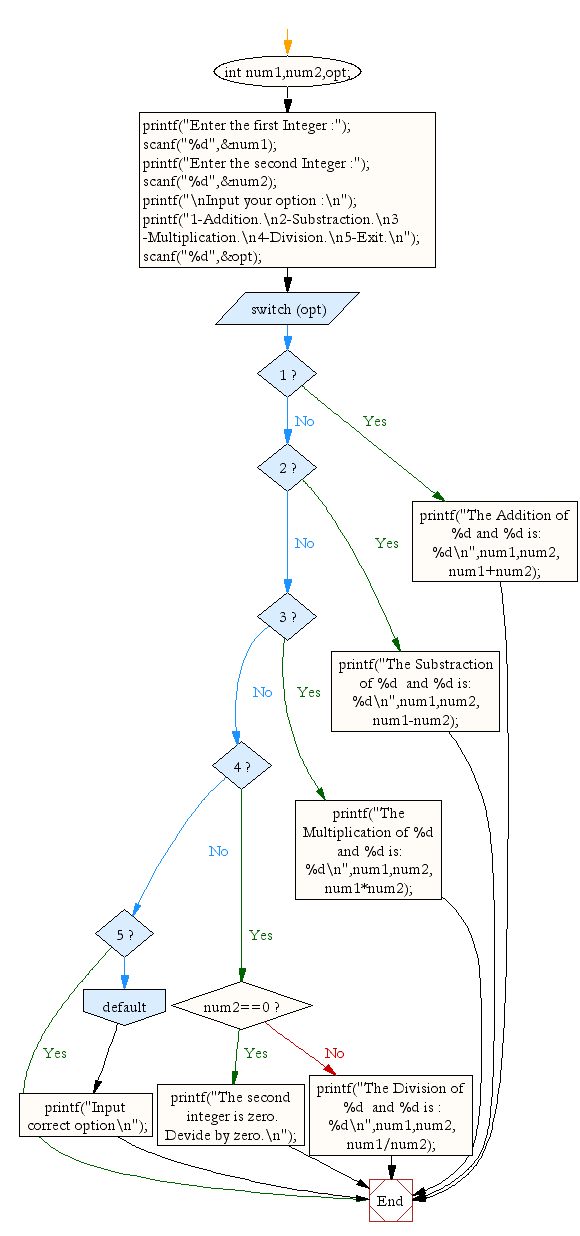﻿ C Program: A menu-driven program for a simple calculator - w3resource# C Exercises: A menu-driven program for a simple calculator

## C Conditional Statement: Exercise-26 with Solution

Write a program in C which is a Menu-Driven Program to perform a simple calculation.

Pictorial Presentation:Sample Solution:

C Code:

``````#include <stdio.h>
void main() {
int num1,num2,opt;
printf("Enter the first Integer :");
scanf("%d",&num1);
printf("Enter the second Integer :");
scanf("%d",&num2);

scanf("%d",&opt);
switch(opt) {
case 1:
printf("The Addition of  %d and %d is: %d\n",num1,num2,num1+num2);
break;

case 2:
printf("The Substraction of %d  and %d is: %d\n",num1,num2,num1-num2);
break;

case 3:
printf("The Multiplication of %d  and %d is: %d\n",num1,num2,num1*num2);
break;

case 4:
if(num2==0) {
printf("The second integer is zero. Devide by zero.\n");
} else {
printf("The Division of %d  and %d is : %d\n",num1,num2,num1/num2);
}
break;

case 5:
break;

default:
printf("Input correct option\n");
break;
}
}
```
```

Sample Output:

```Enter the first Integer :10
Enter the second Integer :2

2-Substraction.
3-Multiplication.
4-Division.
5-Exit.
3
The Multiplication of 10  and 2 is: 20
```

Flowchart:C Programming Code Editor:

Improve this sample solution and post your code through Disqus.

What is the difficulty level of this exercise?

Test your Programming skills with w3resource's quiz.

﻿

## C Programming: Tips of the Day

Why do C and C++ compilers allow array lengths in function signatures when they're never enforced?

It is a quirk of the syntax for passing arrays to functions.

Actually it is not possible to pass an array in C. If you write syntax that looks like it should pass the array, what actually happens is that a pointer to the first element of the array is passed instead.

Since the pointer does not include any length information, the contents of your [] in the function formal parameter list are actually ignored.

Ref : https://bit.ly/3fhlvdH